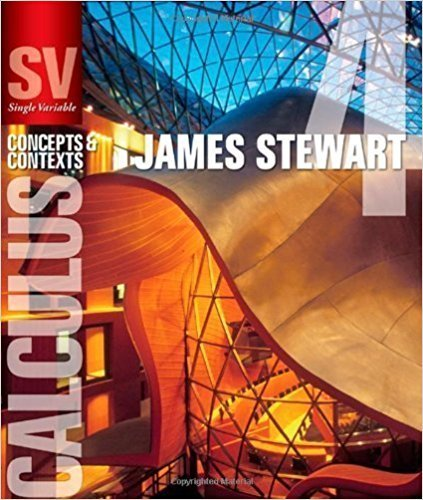×
×

# Solutions for Chapter 6: APPLICATIONS OF INTEGRATION## Full solutions for Single Variable Calculus: Concepts and Contexts (Stewart's Calculus Series) | 4th Edition

ISBN: 9780495559726Solutions for Chapter 6: APPLICATIONS OF INTEGRATION

Solutions for Chapter 6
4 5 0 362 Reviews
15
4
##### ISBN: 9780495559726

Since 39 problems in chapter 6: APPLICATIONS OF INTEGRATION have been answered, more than 20078 students have viewed full step-by-step solutions from this chapter. This textbook survival guide was created for the textbook: Single Variable Calculus: Concepts and Contexts (Stewart's Calculus Series), edition: 4. This expansive textbook survival guide covers the following chapters and their solutions. Chapter 6: APPLICATIONS OF INTEGRATION includes 39 full step-by-step solutions. Single Variable Calculus: Concepts and Contexts (Stewart's Calculus Series) was written by and is associated to the ISBN: 9780495559726.

Key Calculus Terms and definitions covered in this textbook
• Additive inverse of a complex number

The opposite of a + bi, or -a - bi

• Combinations of n objects taken r at a time

There are nCr = n! r!1n - r2! such combinations,

• Continuous at x = a

lim x:a x a ƒ(x) = ƒ(a)

• Difference of complex numbers

(a + bi) - (c + di) = (a - c) + (b - d)i

• Double inequality

A statement that describes a bounded interval, such as 3 ? x < 5

• Fibonacci numbers

The terms of the Fibonacci sequence.

• Inverse reflection principle

If the graph of a relation is reflected across the line y = x , the graph of the inverse relation results.

• Line of symmetry

A line over which a graph is the mirror image of itself

• Linear correlation

A scatter plot with points clustered along a line. Correlation is positive if the slope is positive and negative if the slope is negative

• Local maximum

A value ƒ(c) is a local maximum of ƒ if there is an open interval I containing c such that ƒ(x) < ƒ(c) for all values of x in I

• n-set

A set of n objects.

• Octants

The eight regions of space determined by the coordinate planes.

• Parallel lines

Two lines that are both vertical or have equal slopes.

• Parametric equations for a line in space

The line through P0(x 0, y0, z 0) in the direction of the nonzero vector v = <a, b, c> has parametric equations x = x 0 + at, y = y 0 + bt, z = z0 + ct.

• Reflection

Two points that are symmetric with respect to a lineor a point.

• Second

Angle measure equal to 1/60 of a minute.

• Series

A finite or infinite sum of terms.

• Symmetric matrix

A matrix A = [aij] with the property aij = aji for all i and j

• Union of two sets A and B

The set of all elements that belong to A or B or both.

• z-axis

Usually the third dimension in Cartesian space.

×